Get Started »

# Variables & Functions

(It’s best to start with lowercase letters, reserving capitals for built-in objects.)
 In:=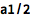⨯ `a1/2`
 Out=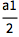A space between two variables or numbers indicates multiplication:

(In other words, “a b” is a times b, whereas “ab” is the variable ab.)
 In:=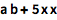⨯ `a b + 5 x x`
 Out=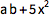Use /. and `` to make substitutions in an expression:

(The “rule” `` can be typed as ->.)
 In:=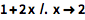⨯ `1 + 2 x /. x -> 2`
 Out=Assign values using the = symbol:

 In:=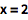⨯ `x = 2`
 Out=Use your variable in expressions and commands:

 In:=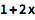⨯ `1 + 2 x`
 Out=Clear the assignment, and x remains unevaluated:

 In:=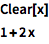⨯ ```Clear[x] 1 + 2 x```
 Out=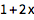Define your own functions with the construction f[x_]:=

 In:=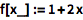⨯ `f[x_] := 1 + 2 x`

x_ means that x is a pattern that can have any value substituted for it.

:= means that any argument passed to f is substituted into the right-hand side upon evaluation:

 In:=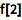⨯ `f`
 Out=QUICK REFERENCE: Defining Variables and Functions `»`